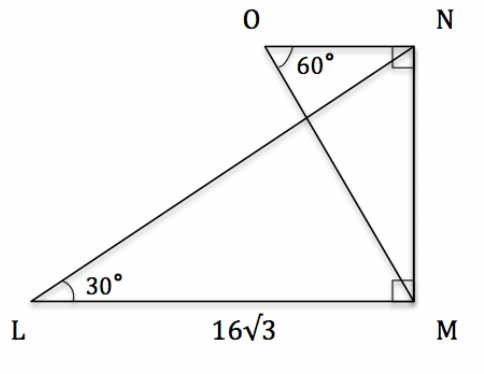# ACT Math Quizzes Online, Trivia

This is an MCQ-based quiz on the topic of ACT Math Quizzes Online, Trivia.

This set of MCQs helps you brush up on the important mathematics topic and prepare you to dive into skill practice and expand your knowledge to tackle the question and answer it carefully.

Start Quiz

If we have a regular (the triangles are equilateral) triangular prism of volume 32in3 and the side length of the triangle on either face is 3in, what is the length of the prism? Write your answer in terms of a decimal rounded to the nearest hundredth.

12.32

14.23

15.46

18.90

Solve for x in the follwing equation:

91x=7,735

88

85

86

84

Solve:  11+13+15

19

39

49

59

Write  27/25 as a percent

78

102

108

46

The length, in meters, of a rectangular fence is 4 more than twice its width. Which of the following gives the length (l)  in terms of width (w) of the rectangular fence?

l = 2w + 4

l = 2w + 24

l = 2w + 10

l = 2w + 8

For the triangles in the figure given, which of the following is closest to the length of line NO?7

6

9

10

Consider a right triangle with an inner angle x(x<90∘).

If

cosx=35

and

sinx=45

what is tanx?

5

1/5

4/3

1

Circle A is centered about the origin and has a radius of 5. What is the equation of the line that is tangent to Circle A at the point (–3,4)?

3x – 4y = –25

3x + 4y = –25

3x – 4y = 25

3x – 4y = 30

Given the following equation:

y = –3x – 5

What is y when x = –2?

3

2

1

-1

There are 300 sandwiches at a company-wide picnic. If half of the sandwiches are tuna, and the provider mistakenly uses expired tuna in three-fifths of those sandwiches, what percentage of total sandwiches still remain edible?

40

70

60

30

Quiz/Test Summary
Title: ACT Math Quizzes Online, Trivia
Questions: 10
Contributed by: